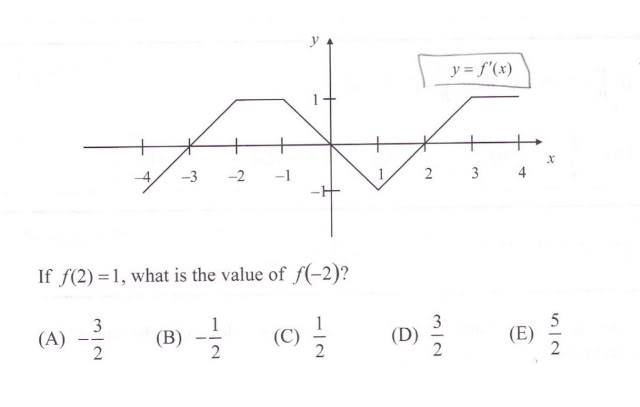# Evaluating point using derivative graph

## Homework Statement*note that this is the graph of the derivitive of the function f(x).

## Homework Equations

Possibly the fundamental theorems of calculus?

## The Attempt at a Solution

I know this problem should be easy, but I am not seeing how my book comes to the answer. Below is the solution my book gives:
f(-2)=f(2) + $$\int$$f'(x)dx = 1-$$\frac{1}{2}$$
The limits of integration are from 2 to -2

Obviously, the first part of the eqn is given, but what exactly does the integral do, and why should the limits be reversed? Furthermore, it seems that if you evaluated the eqn, you would end up with:
f(2) + f(-2) - f(2)
it seems like this would get you nowhere. . .

Last edited:

Dick
Homework Helper
Well, first of all what is the integral of f'(x) from -2 to 2? Just add up the signed area under the curve of f'(x). Then use that the result should be f(2)-f(-2) by the fundamental theorem of calculus.

LCKurtz
Homework Helper
Gold Member
You know the fundamental theorem of calculus, if F is an antiderivative of f then

$$F(b) - F(a) = \int_a^b f(x)\, dx$$

The point being that the integrand, f, is the derivative of the function F. Rephrasing it in those terms gives the equivalent form

$$f(b) - f(a) = \int_a^b f'(x)\, dx$$

Using -2 and 2 for a and b:

$$f(2) - f(-2) = \int_{-2}^2 f'(x)\, dx$$

and solving for f(-2) gives your equation

$$f(-2) = f(2) - \int_{-2}^2 f'(x)\, dx$$

Now to solve the problem you have only to realize that integrals geometrically represent signed area under the curve. So look at the picture and total up that area.

Oh, I was unaware of the equivalent form. This makes much more sense now!

One last question though. One of the theorems states that the derivative of an integral is the original function. Does this statement also work in reverse? I.e. the integral of a derivative also yields the original equation?

Dick
Homework Helper
Oh, I was unaware of the equivalent form. This makes much more sense now!

One last question though. One of the theorems states that the derivative of an integral is the original function. Does this statement also work in reverse? I.e. the integral of a derivative also yields the original equation?

Yes, the integral of a derivative is the difference of the original function.

LCKurtz